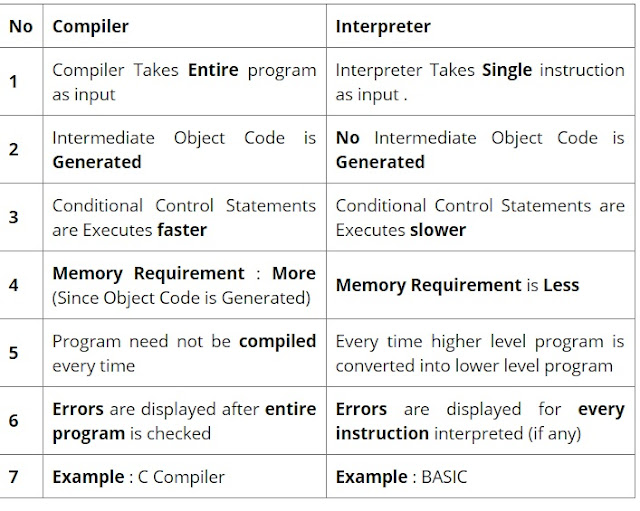L8: Programming For Problem Solving ,What is Algorithm, Computer Algorithm, Largest of three Number1.8 Algorithm
The word algorithm comes from the name of a Persian author, Abu Ja’far Mohammed ibn Musa al Khowarizmi (c. 825 A.D.), who wrote a textbook  on mathematics. This word has taken on a special significance in computer science, where “algorithm” has come to refer to a method that can be used  by a computer for the solution of a problem.
An algorithm is a finite set of instructions that,  if followed, accomplishes a particular task. In addition, all algorithms must  satisfy the following criteria:
1. Input. Zero or more quantities are externally supplied.
2. Output. At least one quantity is produced.
3. Definiteness. Each instruction is clear and unambiguous.
4. Finiteness. If we trace out the instructions of an algorithm, then for  all cases, the algorithm terminates after a finite number of steps.
5. Effectiveness. Every instruction must be very basic so that it can be  carried out, in principle, by a person using only pencil and paper.
Example1 : Algorithm to add two numbers

 Step 1: Start Step 2: Declare variables num1, num2 and sum. Step 3: Read values num1 and num2. Step 4: Add num1 and num2 and assign the result to sum.         sum←num1+num2 Step 5: Display sum Step 6: Stop

Example 2: Write an algorithm to find the largest among three different numbers

 Step 1: Start Step 2: Declare variables a,b and c. Step 3: Read variables a,b and c. Step 4: If a>b            If a>c               Display a is the largest number.            Else               Display c is the largest number.         Else            If b>c               Display b is the largest number.            Else               Display c is the greatest number.  Step 5: Stop

L7:Programming Languages,Difference between compiler & interpreter,Compiler vs Interpreter in HindiThere are two general methods by which a program can be
executed. It can be compiled, or it can be interpreted. Although programs written in any computer
language can be compiled or interpreted, some languages are designed more for one form of
execution than the other. For example, Java was designed to be interpreted, and C was designed to
be compiled